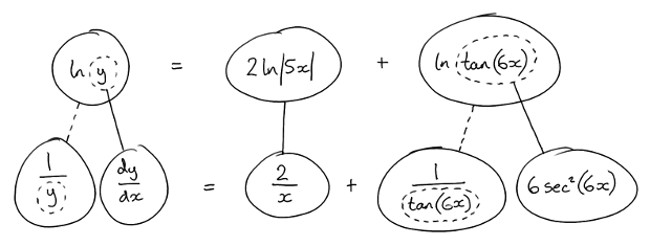# Thread: Logarithmic Differentiation with binomial products

1. ## Logarithmic Differentiation with binomial products

Hi,

I've been asked to differentiate $\displaystyle y = 5x^2tan(6x)$ logarithmically.

I took the following steps:

$\displaystyle y = 5x^2tan(6x)$

$\displaystyle ln(y) = ln(5x^2tan6x) = (2ln5x)(lntan(6x))$

$\displaystyle f(x) = 2ln5x$
$\displaystyle g(x) = lntan(6x)$
$\displaystyle f'(x) = \frac {2}{x}$
$\displaystyle g'(x) = \frac {1}{6Sec^26x}$

$\displaystyle \frac {1}{y} \frac{dy}{dx} = (2ln5x)(\frac{1}{6Sec^26x})+(\frac{2}{x})(lntan(6x ))$

$\displaystyle \frac {dy}{dx} = y((2ln5x)(\frac{1}{6Sec^26x})+(\frac{2}{x})(lntan( 6x)))$

$\displaystyle \frac{dy}{dx} = 5x^2tan(6x) ((2ln5x)(\frac{1}{6Sec^26x})+(\frac{2}{x})(lntan(6 x)))$

This is my first attempt at differentiating binomial products logarithmically, can anyone please look at these steps and tell me if and how I've gone wrong?

Thanks.

(Please spare any factorising, I don't understand it very well right now)

2.Originally Posted by ashleysmithd$\displaystyle y = 5x^2tan(6x)$

$\displaystyle ln(y) = ln(5x^2tan6x) = (2ln5x)(lntan(6x))$
The log of a product is a sum of two logs - not a product of 2 logs.Originally Posted by ashleysmithd$\displaystyle f(x) = 2ln5x$
$\displaystyle g(x) = lntan(6x)$
$\displaystyle f'(x) = \frac {2}{x}$
$\displaystyle g'(x) = \frac {1}{6Sec^26x}$
So you don't want the product rule, though you do want to differentiate f and g. Your f is fine, but g dodgy.

Just in case a picture helps...... where (key in spoiler) ...

Spoiler:... is the chain rule. Straight continuous lines differentiate downwards (integrate up) with respect to the main variable (in this case ), and the straight dashed line similarly but with respect to the dashed balloon expression (the inner function of the composite which is subject to the chain rule).

_________________________________________

Don't integrate - balloontegrate!

Balloon Calculus; standard integrals, derivatives and methods

Balloon Calculus Drawing with LaTeX and Asymptote!

3. $\displaystyle \ln(y) = \ln(5) + 2\ln(x) + \ln(\tan(6x))$

The first two terms are simple enough to differentiate but you will need to use the chain rule twice on the last term

Let $\displaystyle u = tan(6x) \implies \dfrac{du}{dx} = 6\sec^2(6x)$ and also gives $\displaystyle y = \ln(\tan(6x)) = \ln(u)$ and by the chain rule $\displaystyle \dfrac{dy}{dx} = \dfrac{dy}{du} \cdot \dfrac{du}{dx}$

(note that y here is used as an example to demonstrate the chain rule)

4. I think I see what you mean, g(x) was a function of a function and needed the chain rule to differentiate it into: $\displaystyle (\frac{1}{tan(6x)}(6sec^26x))$ ?

I'm not quite what you meant by using the chain rule twice, I've equated it to this so far:
$\displaystyle \frac{dy}{dx} = 5x^2tan6x ((2ln5x)(\frac{1}{tan(6x)}(6sec^26x))+(lntan6x)(\f rac{2}{x}))$

Is this on the right lines?

5.Originally Posted by ashleysmithd$\displaystyle y = 5x^2tan(6x)$ logarithmically.
Why make it so complicated?
The derivative is simply: $\displaystyle 10x\tan(6x)+30x^2\sec^2(6x)$.

6. I completely understand it can be factorised down, but I really don't understand factorising well yet so I'd rather work long hand and just get the rules of differentiation right first.
That said, all be it unnecessarily long, is the solution I posted above correct?

7. It is a simple product: $\displaystyle 5x^2$ times $\displaystyle \tan(6x)$.

Use the product rule. Anything else is a waste of time and effort.

8. I would do but the point of the exercise I've given is to learn how to differentiate logarithmically, and show working, so I can't use the product rule on its own annoyingly.

9.Originally Posted by ashleysmithdI think I see what you mean, g(x) was a function of a function and needed the chain rule to differentiate it into: $\displaystyle (\frac{1}{tan(6x)}(6sec^26x))$ ?
Good.

I'm not quite what you meant by using the chain rule twice, I've equated it to this so far:
$\displaystyle \frac{dy}{dx} = 5x^2tan6x ((2ln5x)(\frac{1}{tan(6x)}(6sec^26x))+(lntan6x)(\f rac{2}{x}))$

Is this on the right lines?
No, it looks like you're still trying to involve the product rule even though the one advantage of doing it logarithmically (although, as Plato says, this example is not remotely a good case for that treatment) is that you got rid of the product so you don't need that rule.

Look at the bottom row of my diagram and multiply both sides by y, perhaps?

10. I think the part where I'm getting confused is how
$\displaystyle ln(y) = ln(5x^2tan6x)$
becomes
$\displaystyle ln(y) = 2ln5x + lntan6x$

I don't understand why these functions are being added and not multiplied? Why does it become a sum, and not remain a product, is it to do with log properties?

11.Originally Posted by ashleysmithdis it to do with log properties?
Yes - revise them.

$\displaystyle \ln(ab)\ =\ \ln(a) + \ln(b)\ \ \$

from

$\displaystyle a = e^x$ and $\displaystyle b = e^y$

and

$\displaystyle ab = e^{x+y}$

12.Originally Posted by ashleysmithdI think the part where I'm getting confused is how
$\displaystyle ln(y) = ln(5x^2tan6x)$
becomes
$\displaystyle ln(y) = 2ln5x + lntan6x$
It doesn't - you can only take a power out when each term is raised to the power. You moved the 2 to the front but only the x was squared, not the 5

$\displaystyle \ln(y) = \ln(5x^2 \tan(6x)) = \ln(5x^2) + \ln(\tan(6x))$

You need to use the addition rule on $\displaystyle \ln(5x^2)$ before the power rule can be done on x^2 -> $\displaystyle \ln(5x^2) = \ln(5) + \ln(x^2) = \ln(5) + 2\ln(x)$

Hence $\displaystyle \ln(y) = \ln(5) + 2\ln(x) + \ln(\tan(6x))$

Use the chain rule (explained in posts 2 and 3) to differentiate $\displaystyle \ln(\tan(6x))$

13. I you want to learn Logarithmic Differentiation then do right.
Suppose that each of $\displaystyle g,~h,~j,~\&~k$ is a differentiable function and $\displaystyle f(x)=g(x)\cdot h(x)\cdot j(x)\cdot k(x)\cdot$.
Then the derivative is $\displaystyle f'(x) = f(x)\left[ {\frac{{h'(x)}}{{h(x)}} + \frac{{g'(x)}}{{g(x)}} + \frac{{j'(x)}}{{j(x)}} + \frac{{k'(x)}}{{k(x)}}} \right]$.
Not that can be done for any numbers of factors.

So the derivative of $\displaystyle 5x^2\tan(6x)$ is simply $\displaystyle 5x^2 \tan (5x)\left[ {\frac{2}{{x^2 }} + \frac{{6\cos (6x)}}{{\sin (6x)}} + \frac{{6\sin (6x)}}{{\cos (6x)}}} \right]$.

But this is a horrible example to teach that concept.

14. Ok thanks, I think I just got caught out on the log rules.